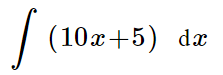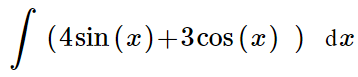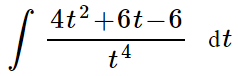### Day 50 - Area Under a Curve - 10.28.14

 UpdatesUnit 4 Test will be on Friday, 11/14!Bell RingerAntiderivativeFind the following5x2 + 5x + C10x2 + 5x + C5x2 + 5x10none of the aboveFind the following4cos(x) - 3sin(x) + C-4cos(x) + 3sin(x)-4cos(x) - 3sin(x) + C-4cos(x) + 3sin(x) + C-3cos(x) + 4sin(x) + CFind the following4/t - 3/t2 + 2/t3 + C-4/t - 3/t2 + 2/t3 + C-4/t + 3/t2 + 2/t3-4/t - 3/t2 + 2/t3-4/t + 6/t2 + 6/t3 + CFind F(x) if F’(x) = -6x2 - 3 given F(2) = -3F(x) = -2x3 + 19F(x) = -2x3 + CF(x) = -12x3 - 3x + 19F(x) = -2x3 - 3x + 19F(x) = -2x3 + 3xReviewPrerequisitesDerivativesDerivative RulesPower RuleConstant RuleTrig DerivativesSigma NotationAntiderivativeIndefinite IntegralDefinitionIntegral SignIntegrandVariable of IntegrationConstant of IntegrationGeneral SolutionBasic Integration RulesLessonArea Under a CurveQuestion InputCheckpointsExit TicketPosted on the board at end of the block. Lesson Objective(s)How can the area under a curve be approximated?How can the area under a curve be calculated?Standard(s)APC.10Use Riemann sums and the Trapezoidal Rule to approximate definite integrals of functions represented algebraically, graphically, and by a table of values and will interpret the definite integral as the accumulated rate of change of a quantity over an interval interpreted as the change of the quantity over the intervalRiemann sums will use left, right, and midpoint evaluation points over equal subdivisions.APC.11​The student will find antiderivatives directly from derivatives of basic functions and by substitution of variables (including change of limits for definite integrals).APC.12​The student will identify the properties of the definite integral. This will include additivity and linearity, the definite integral as an area, and the definite integral as a limit of a sum as well as the fundamental theorem.APC.13​The student will use the Fundamental Theorem of Calculus to evaluate definite integrals, represent a particular antiderivative, and facilitate the analytical and graphical analysis of functions so defined.APC.14​The student will find specific antiderivatives, using initial conditions (including applications to motion along a line). Separable differential equations will be solved and used in modeling (in particular, the equation y' = ky and exponential growth).APC.15​The student will use integration techniques and appropriate integrals to model physical, biological, and economic situations. The emphasis will be on using the integral of a rate of change to give accumulated change or on using the method of setting up an approximating Riemann sum and representing its limit as a definite integral. Specific applications will includea)​ the area of a region;b)​ the volume of a solid with known cross-section;c)​ the average value of a function; andd) ​the distance traveled by a particle along a line.Mathematical Practice(s)#1 - Make sense of problems and persevere in solving them#2 - Reason abstractly and quantitatively#5 - Use appropriate tools strategically#6 - Attend to precision#8 - Look for and express regularity in repeated reasoningPast CheckpointsAntiderivativeCheckpoints